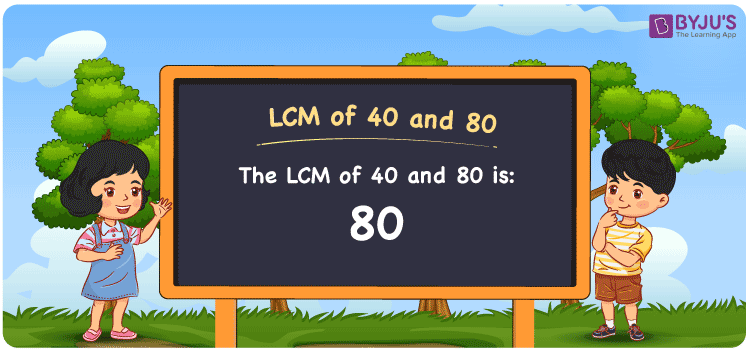# LCM of 40 and 80

LCM of 40 and 80 is 80. 80 is the first multiple that appears in the multiplication table of both 40 and 80. There are abundant multiples that are common to 40 and 80. However, 80 will be the first number that is common. Hence it is the LCM, Least Common multiple or Lowest common multiple. LCD (Least Common Divisor) and LCM are interchangeable terms. Look for more on LCM.

## What is LCM of 40 and 80

The Least Common Multiple or Lowest Common Multiple of 40 and 80 is 80.## How to Find LCM of 40 and 80?

LCM of 40 and 80 can be determined using three methods:

• Prime Factorisation
• Division method
• Listing the multiples

### LCM of 40 and 80 Using Prime Factorisation Method

In the Prime Factorisation method, express the numbers as the product of prime numbers.

Here, 40 and 80 can be expressed as:

40 = 2 × 2 × 2 × 5

80 = 2 × 2 × 2 × 2 × 5

LCM (40, 80) = 2 × 2 × 2 × 2 × 5 = 80

### LCM of 40 and 80 Using Division Method

In the Division Method, the given set of numbers are divided by a smallest number, usually prime, that divides all, until no further division is possible as only 1 is left as the remainder.

 2 40 80 2 20 40 2 10 20 2 5 10 5 5 5 × 1 1

LCM (40, 80) = 2 × 2 × 2 × 2 × 5 = 80

### LCM of 40 and 80 Using Listing the Multiples

By listing all the multiples of given numbers, we can identify a multiple that appears first in both the list and that becomes the LCM. Below is the list of multiples for 40 and 80

 Multiples of 40 Multiples of 80 40 80 80 160 120 240

LCM (40, 80) = 80

## Video Lesson on Applications of LCM## Solved Examples

What is the first number that is divisible by both 40 and 80?

Answer: 80 is the smallest number that is divisible by both 40 and 80.

What is the LCM for 5, 10, 40 and 80?

Answer: LCM for 5, 10, 40 and 80 is 80 as 5 and 10 are the factors of 40 and 80.

## Frequently Asked Questions on LCM of 40 and 80

### What is the LCM of 40 and 80?

The LCM of 40 and 80 is 80.

### Is the Least Common Multiple of 40 and 80 the same as the Highest Common Factor of 40 and 80?

No. LCM of 40 and 80 is 80 and HCF of 40 and 80 is 40.

### What are the common prime factors of 40 and 80?

2 and 5 are the common prime factors of 40 and 80.

### What are the first 3 common multiples of 40 and 80?

The first three common multiples of 40 and 80 are 80, 160, and 240.

### Is the LCM of 4 and 40 same as the LCM of 8 and 80?

No. LCM of 4 and 40 is 40, whereas the LCM of 8 and 80 is 80. They are not the same.